Formula · Tabular Method · u-v Method · Examples · Exercises · Advanced · Derivation
Keywords · Navigation · Recommended Books · Site Index · Gift ShopIntegration By Parts

Suppose we have two functions multiplied by each otherand differentiate according to the product rule:then by integrating both sides between the limits a and band rearranging givesor as the formula is better knownINTEGRATION BY PARTS

Tricks: If one of the functions is a polynomial (say nth order) and the other is integrable n times, then you can use the fast and easy Tabular Method:Tabular Method
Supposeand. Then if we set up a table, differentiating f(x) as many times as it takes to get to zero and integrating g(x) as many times, we get
 D I(a)(b) +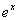(a)(c) -(b)+(c)
- notice how we alternate the signs in the “I” column. Then, multiplying rows with like letters (which you can skip on your own paper to help make this clearer - draw arrows instead from (a) to (a), etc.) gives the following directly as the anti-derivative:This method is much faster than the f-g method or the older u-v, especially for iterated (more than once) integrals by parts (Thanks to Dr. William T. Guy, UT Austin).Advanced

There is a way to extend the tabular method to handle arbitrarily large integrals by parts - you just include the integral of the product of the functions in the last row and pop in an extra sign (whatever is next in the alternating series), so thatThe trick is to know when to stop for the integral you are trying to do. Try it for a few simple functions, you'll see!The u - v Method:

This is the old standbywhich is identical to the canonical method if you setMany find this a lot easier to remember!Examples || 1 || 2 || 3 ||

Example 1D I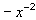So according to the table,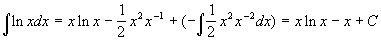With the u - v method,andup

Example 2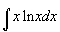D Ix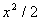So according to the table,up

Example 3D I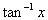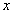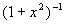This one is a bit more fun… from the table we haveThe last integral on the right yields to the substitutionand, viz.which makes the final integralup

So this method can be used for even very nasty integrals!Exercises || 1 || 2 || 3 ||
These are really proofs since I give you the answers.

Use the above methods to show

1.2.3.Recommended Books
The classic calculus problem book - very light on theory, plenty of problems with full solutions, more problems with answers

A simplified and updated version of the classic Schaum's Outline. Not as complete as the previous book, but enough for most studentsKeywords

integral, integration, technique, parts, products, tabular, u-v